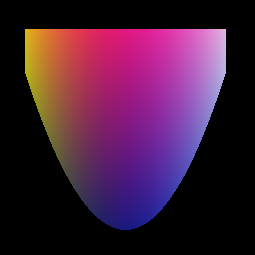Fib multimedia objects can be directly stored in a readable form in the XML-format (see documentation section XML format).

For a better understanding of the Fib multimedia format and to illustrate its possibilities, a relatively simple example for Fib is given below.

## CrestThe picture, for the example, is shown on the left. It is a crest-shaped object with a color gradient. The image size is 255 times 255 pixels in RGB color.

The XML description in Fib of the image is given below. For better readability it is presented somewhat excessively.

The Fib object in the compressed Fib storage format is structurally the same, but it is stored more efficiently.
In the compressed Fib storage format the image requires only 153 bytes, of which 134 bytes are used for setting up the environment (the root-element, without the "main_fib_object"-element), that means, only 19 bytes are needed for the actual presentation of the crest.

• Fib format: 153 bytes, of which 134 bytes are for environment and 19 bytes are for the crest
• Fib XML format: around 1,900 bytes (with indentation and line breaks; see below)
• PNG format: 2,500 bytes
• JPG format: 4,720 bytes (using GIMP: Quality 85, optimized smoothing 0)
• BMP format: 196,000 bytes

### XML representation of the image

``` <?xml version="1.0" encoding="UTF-8"?> <fib_object xmlns:xsd="http://www.w3.org/2001/XMLSchema-instance" xmlns="http://www.fib-development.org/" xsd:schemaLocation="http://www.fib-development.org/fib.xsd"> <root> <domains> <dim count="2"> <dimension number="1" direction="horizontal"/> <dimension number="2" direction="vertical"/> <vector elements="2"> <naturalNumber max="255" /> <naturalNumber max="255" /> </vector> </dim> <property name="colorRGB" > <vector elements="3"> <naturalNumber max="255" /> <naturalNumber max="255" /> <naturalNumber max="255" /> </vector> </property> <area> <vector elements="2"> <naturalNumber max="1" /> <vector elements="2"> <naturalNumber max="255" /> <naturalNumber max="255" /> </vector> </vector> </area> <subfunction> <naturalNumber max="255" /> </subfunction> </domains> <main_fib_object> <area define_variable="1"> <vector type="subarea"> <value>25</value> <value>225</value> </vector> <function define_variable="2"> <subfunction> <add> <value>25</value> <exp> <div> <sub> <variable>1</variable> <value>125</value> </sub> <value>8</value> </div> <value>2</value> </exp> </add> </subfunction> <area define_variable="3"> <vector type="subarea"> <value>225</value> <variable>2</variable> </vector> <property> <vector type="property.colorRGB"> <variable>3</variable> <variable>2</variable> <variable>1</variable> </vector> <point> <vector type="position"> <variable>1</variable> <variable>3</variable> </vector> </point> </property> </area> </function> </area> </main_fib_object> </root> </fib_object> ```

### Explanations for the XML representation

The root-element defines the environment of the image. It contains two main parts: the specification of the different domains (`<domains>`) and the actual representing Fib object (`<main_fib_object>`).

The domains set the size of the image and numbers, which can be used in the elements.

The Fib object represents a crest-shaped object.
The first area element (`area`) ensures that the first variable (`define_variable="1"`) runs from 25 till 225 (this means it takes the values 25, 26, 27, ..., 225). This variable 1 limits the crest in the horizontal direction (you can see this at the point element `point`).
In the vertical direction the crest is limited by the variable 3. It runs for each assignment to variable 1 from 225 (upper border of the crest) to a (smaller) value, which is evalued by a function (`function`) of variable 1 f(V1). This function is f(V1)=25 + ( (V1 - 125) / 8 )2 . Thus the lower border of the crest is defined by a quadratic function.

The color gradient in the crest is simply generated by the fact, that the available variables are used as color components. Likewise for the color components other functions or fixed numbers could be used.

This XML representation can be converted directly into the image shown above (with the crest) by the Fib converter (convertFromFib).
This makes it possible to directly create Fib representations with an text editor. Necessary for this are only a little practice with writing in the XML-format and knowledge of the Fib XML-elements.